# MCQ Questions for Class 12 Chemistry Chapter 11 Alcohols, Phenols and Ethers with Answers

Students are advised to practice the NCERT MCQ Questions for Class 12 Chemistry Chapter 11 Alcohols, Phenols and Ethers with Answers Pdf free download is available here. MCQ Questions for Class 12 Chemistry with Answers are prepared as per the Latest Exam Pattern. Students can solve these Alcohols, Phenols and Ethers Class 12 MCQs Questions with Answers and assess their preparation level.

## Alcohols, Phenols and Ethers Class 12 MCQs Questions with Answers

Solving the Alcohols, Phenols and Ethers Multiple Choice Questions of Class 12 Chemistry Chapter 11 MCQ can be of extreme help as you will be aware of all the concepts. These MCQ Questions on Alcohols, Phenols and Ethers Class 12 with answers pave for a quick revision of the Chapter thereby helping you to enhance subject knowledge. Have a glance at the MCQ of Chapter 11 Chemistry Class 12 and cross-check your answers during preparation.

Question 1.
Dehydration of tertiary alcohols with Cu at 573 gives
(a) Aldehydes
(b) Ketones
(c) Alkenes
(d) None of these

Answer

Answer: (c) Alkenes

Question 2.
Molecular formula of ethers is:
(a) CnH2n+2O
(b) CnH2nO
(c) CnH2n+1O
(d) None of these

Answer

Answer: (a) CnH2n+2O

Question 3.
Williamson’s synthesis is an example of:
(a) Nucleophilic substitution reaction
(b) Nucleophilic addition
(c) Electrophilic substitution
(d) None of the above

Answer

Answer: (a) Nucleophilic substitution reaction

Question 4.
Reaction used for the preparation of ethers is
(a) Reimer-Tiemann reaction
(b) Williamson’s synthesis
(c) Wurtz reaction
(d) Cannizzaro reaction

Answer

Answer: (b) Williamson’s synthesis

Question 5.
The test used to distinguish alcohols from one another is known as
(a) Hinsberg’s test
(b) 2, 4-DNP test
(c) Iodoform test
(d) Lucas Lest

Answer

Answer: (d) Lucas Lest

Question 6.
The IUPAC name of(a) Propane-1, 3-diol
(b) Propane-1, 2-diol
(c) Propane -1, 2, 3-triol
(d) Glycerol

Answer

Answer: (c) Propane -1, 2, 3-triol

Question 7.
Ethers on hydrolysis give
(a) carboxylic acid
(b) alcohol
(c) ester
(d) ketone

Answer

Answer: (b) alcohol

Question 8.
Which of the following has highest boiling point?
(a) Methanol
(b) Ethanol
(c) Propan-1 -ol
(d) Butan-1-ol

Answer

Answer: (d) Butan-1-ol

Question 9.
Which has highest value of pKa?
(a) Phenol
(b) Ethanol
(c) o-Nitrophenol
(d) o-Cresol

Answer

Answer: (b) Ethanol

Question 10.
Which of the following is most acidic?
(a) Benzyl alcohol
(b) Cyclohexanol
(c) Phenol
(d) m-Chlorophenol.

Answer

Answer: (d) m-Chlorophenol.

Question 11.
Phenols on reaction with cone. HNO3 in the presence of cone. H2SO4 give
(a) o-nitrophenol
(b) m-nitrophenol
(c) p-nitrophenol
(d) 2,4,6-trinitrophenol.

Answer

Answer: (d) 2,4,6-trinitrophenol.

Question 12.
Methanol and ethanol can be distinguished by using
(a) Fehling’s test
(b) Iodoform test
(c) Tollen’s test
(d) Carbylamine test.

Answer

Answer: (b) Iodoform test

Question 13.
The IUPAC name of the compound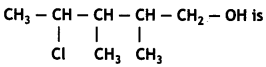(a) 2-Chloro-3,4-dimethyl-n-pentyl alcohol
(b) 2-Chloro-3,4-dimethylpentan-5-ol
(c) 4-Chloro-2,3-dimethylpentan-1 -ol
(d) 2,3-Dimethyl-4-chloropentan-1 -ol.

Answer

Answer: (c) 4-Chloro-2,3-dimethylpentan-1 -ol

Question 14.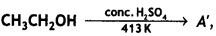A will be
(a) CH2=CH2
(b) C2H5OCH3
(c) (C2H5)2O
(d) CH3CH2CH2CH3

Answer

Answer: (c) (C2H5)2O

Question 15.
Which is weakest acid in the following?
(a) CH3OH
(b) (CH3)2CHOH
(c) CH3CH2OH
(d) (CH3)3COH

Answer

Answer: (a) CH3OH

Question 16.
Williamson synthesis is used to prepare:
(a) Alcohol
(b) Amine
(c) Ketone
(d) Ether

Answer

Answer: (d) Ether

Question 17.
In the reaction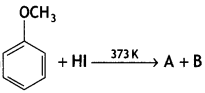A and B are:
(a) C6H5l, CH3OH
(b) C6H5OH, CH3l
(c) C6H5CH2OH, CH3l
(d) CH3CH2l, C6H5OH

Answer

Answer: (b) C6H5OH, CH3l

Question 18.
The IUPAC name of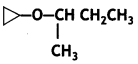(a) 2-Cyclopropoxybutane
(b) 2-Propoxybutane
(c) 2-Propoxypropane
(d) 2-Methyl-2-propoxypropane

Answer

Answer: (a) 2-Cyclopropoxybutane

Question 19.
Neutral ferric chloride test can be used to distinguish between
(a) Alcohols and ethers
(b) Aldehydes and ketones
(c) Amines and aldehyde
(d) Phenols and alcohols

Answer

Answer: (d) Phenols and alcohols

Question 20.
On reaction with aqueous bromine at room temperature, phenol forms which of the following?
(a) meta-Bromophenol
(b) 2, 6-Dibromophenol
(c) 2, 4, 6-Tribromophenol
(d) 3, 5-Dibromophenol

Answer

Answer: (c) 2, 4, 6-Tribromophenol

Question 21.
How many alcohols with molecular formula C4H10O are chiral in nature?
(a) 1
(b) 2
(c) 3
(d) 4

Answer

Answer: (a) 1

Question 22.
What is the correct order of reactivity of alcohols in the following reaction?(a) 1° > 2° > 3°
(b) 1° < 2° > 3°
(c) 3° > 2° > 1°
(d) 3° > 1° > 2°

Answer

Answer: (c) 3° > 2° > 1°

Question 23.
CH3CH2OH can be converted into CH3CHO by
(а) catalytic hydrogenation
(b) treatment with LiAlH4
(c) treatment with pyridinium chlorochromate
(d) treatment with KMnO4

Answer

Answer: (c) treatment with pyridinium chlorochromate

Question 24.
The process of converting alkyl halides into alcohols involves
(a) addition reaction
(b) substitution reaction
(c) dehydrohalogenation reaction
(d) rearrangement reaction

Answer

Answer: (b) substitution reaction

Question 25.
Which of the following compounds is aromatic alcohol?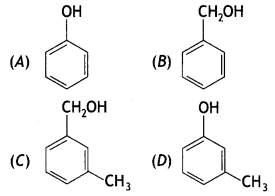(a) A, B, C, D
(b) A, D
(c) B, C
(d) A

Answer

Answer: (c) B, C

Question 26.
Give IUPAC name of the compound given below.(a) 2-Chloro-5-hydroxyhexane
(b) 2-Hydroxy-5-chlorohexane
(c) 5-Chlorohexan-2-ol
(d) 2-Chlorohexan-5-ol

Answer

Answer: (c) 5-Chlorohexan-2-ol

Question 27.
IUPAC name of m-cresol is
(a) 3-methylphenol
(b) 3-chlorophenol
(c) 3-methoxyphenol
(d) benzene-1,3-diol

Answer

Answer: (a) 3-methylphenol

Question 28.
IUPAC name of the compound(a) 1-methoxy-1-methylethane
(b) 2-methoxy-2-methylethane
(c) 2-methoxypropane
(d) isopropylmethyl ether

Answer

Answer: (c) 2-methoxypropane

Question 29.
Which of the following species can act as the strongest base?
(a) ΘOH
(b) ΘOR
(c) ΘOC6H5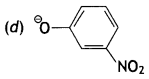Answer

Answer: (b) ΘOR

Question 30.
Which of the following compounds will react with sodium hydroxide solution in water?
(a) C6H5OH
(b) C6H5CH2OH
(c) (CH3)3COH
(d) C2H5OH

Answer

Answer: (a) C6H5OH

Question 31.
Phenol is less acidic than
(a) ethanol
(b) o-nitrophenol
(c) o-methylphenol
(d) o-methoxyphenol

Answer

Answer: (d) o-methoxyphenol

Question 32.
Which of the following is most acidic?
(a) Benzyl alcohol
(b) Cyclohexanol
(c) Phenol
(d) m-Chlorophenol

Answer

Answer: (d) m-Chlorophenol

Question 33.
Mark the correct order of decreasing acid strength of the following compounds.(a) E>D>B>A>C
(b) B>D>A>C>E
(c) D>E>C>B>A
(d) E>D>C>B>A

Answer

Answer: (b) B>D>A>C>E

Question 34.
Mark the correct increasing order of reactivity of the following compounds with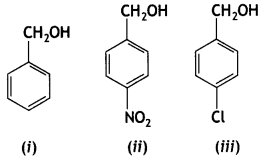(a) (i) < (ii) < (iii)
(b) (ii) < (i) < (iii)
(c) (ii) < (iii) < (i)
(d) (iii) < (ii) < (i)

Answer

Answer: (c) (ii) < (iii) < (i)

Question 35.
Arrange the following compounds in increasing order of boiling point.
Propan-1-ol, butan-1-ol, butan-2-ol, pentan-1-ol
(a) Propan-1-ol, butan-2-ol, butan-1-ol, pentan-1-ol
(b) Propan-1-ol, butan-1-ol, butan-2-ol, pentan-1-ol
(c) Pentan-1-ol, butan-2-ol, butan-1-ol, propan-1 -ol
(d) Pentan-1-ol, butan-1-ol, butan-2-ol, propan-1 -ol

Answer

Answer: (a) Propan-1-ol, butan-2-ol, butan-1-ol, pentan-1-ol

Assertion and Reason Type Questions

The questions given below consist of an assertion and a reason. Use the following key to choose the appropriate answer.
(a) Both Assertion (A) and Reason (R) are correct statements, and Reason (R) is the correct explanation of the Assertion (A).
(b) Both Assertion (A) and Reason (R) are correct statements, but Reason (R) is not the correct explanation of the Assertion (A).
(c) Assertion (A) is correct, Reason (R) is wrong statement.
(d) Assertion (A) is wrong, but Reason (R) is correct statement.

Question 36.
Assertion: C2H5OH is a weaker base than phenol but is a stronger nucleophile than phenol.
Reason: In phenol the lone pair of electrons on oxygen is withdrawn towards the ring due to resonance.

Answer

Answer: (d) Assertion (A) is wrong, but Reason (R) is correct statement.

Question 37.
Assertion: The boiling points of alcohols are higher than those of hydrocarbons of comparable molecular mass.
Reason: Alcohols show intramolecular hydrogen bonding.

Answer

Answer: (c) Assertion (A) is correct, Reason (R) is wrong statement.

Question 38.
Assertion: Phenol undergoes Kolbe’s reaction but ethanol does not.
Reason: Phenol is more acidic than ethanol.

Answer

Answer: (b) Both Assertion (A) and Reason (R) are correct statements, but Reason (R) is not the correct explanation of the Assertion (A).

Question 39.
Assertion: The C-O-C bond angle in ethers is higher than H-O-H bond angle in water.
Reason: Oxygen in both ethers and water is sp³ hybridised.

Answer

Answer: (b) Both Assertion (A) and Reason (R) are correct statements, but Reason (R) is not the correct explanation of the Assertion (A).

Question 40.
Assertion: The boiling point of diethyl ether is much less than that of ethanol.
Reason: In ethanol, the molecules are associated by the formation of intermolecular hydrogen bonding, whereas in diethyl ether, it is not possible.

Answer

Answer: (a) Both Assertion (A) and Reason (R) are correct statements, and Reason (R) is the correct explanation of the Assertion (A).

Question 41.
Assertion: Alcohols have higher boiling points than ethers of comparable molecular masses.
Reason: Alcohols and ethers are isomeric compounds.

Answer

Answer: (b) Both Assertion (A) and Reason (R) are correct statements, but Reason (R) is not the correct explanation of the Assertion (A).

Question 42.
Assertion: o- and p-nitrophenols can be separated by steam distillation.
Reason: o-isomer is steam volatile due to chelation, while p-isomer is not steam volatile due to association of molecules by intermolecular H-bonding.

Answer

Answer: (a) Both Assertion (A) and Reason (R) are correct statements, and Reason (R) is the correct explanation of the Assertion (A).

Hope the information shared regarding the NCERT MCQ Questions for Class 12 Chemistry Chapter 11 Alcohols, Phenols and Ethers with Answers Pdf free download is true and genuine as far as our knowledge is concerned. If you feel any information regarding CBSE Class 12 Chemistry Alcohols, Phenols and Ethers MCQs Multiple Choice Questions with Answers is missing do reach us and we will look into it and add it accordingly.

error: Content is protected !!